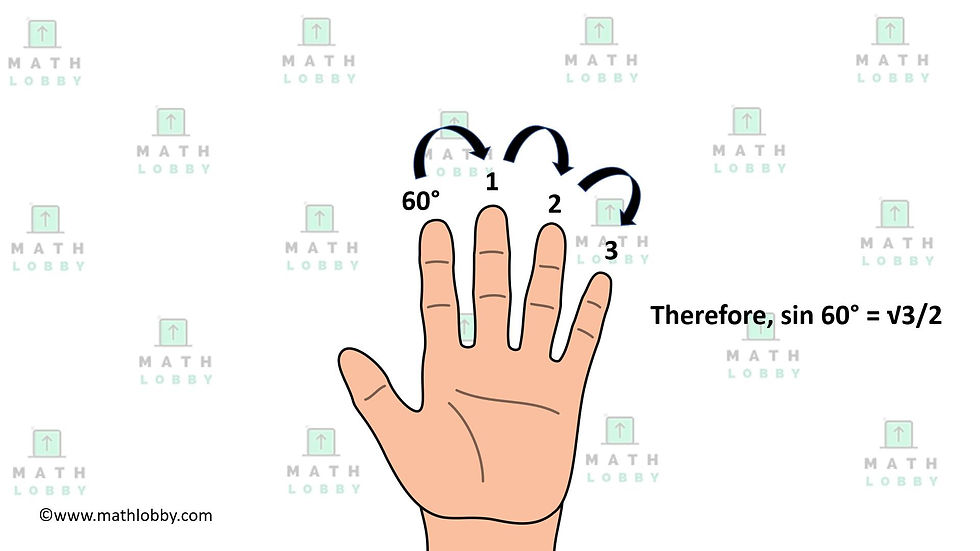top of page
Search

# How To Memorize Trigonometric Functions Using Fingers!

Updated: Jun 22, 2021

Have you ever stressed yourself over memorizing trigonometric functions since there is a whole list to it? Or trying to figure out the fractional answers to make your workings or answers simplified and neater because your calculator always gives you a long string of numbers?

If you are wondering if there is an easier way to go about this, then look no further!

Today, Math Lobby is going to teach you a “math hack” that you can apply, whether you are trying to solve questions in an assignment or save time during examinations. All we need today is just going to be at your fingertips!

This “math hack” allows you to find the exact trigonometric values of very common sine and cosine functions. Let’s begin!

Through this method, you can find the sine and cosine functions of 5 specific angles: 0°,30°,45°,60° and 90°. For this hack, you will only have to memorize one thing, which is the square root of x divided by 2, where x is the number of fingers to the right (regardless for sine or cosine!) of the finger you closed. Let’s see how it’s done!First, hold out your left hand and face it towards you.

For sine functions, your pinky will represent 0°, ring finger will represent 30°, middle finger will represent 45°, index finger will represent 60° and your thumb will represent 90°. For cosine functions, it will be the exact opposite, meaning that instead of your pinky, it will be your thumb that represents 0°, followed by your index finger representing 30°, so on and so forth.

Next, for any trigonometric value you want to find, just close the finger that represents the angle and count the number of fingers to the right for sine functions, and the left for cosine functions!

Let’s see how it’s done:For example, if we want to find the trigonometric value of sin(60°), we must then remember that for sine functions, we have to start from the right. The angle 60° will hence fall on our index finger, which means that we have 3 fingers (middle, ring and pinky) to our right. Therefore, if we apply the rule that we memorized, square root of x divided by 2, which in this case x = 3, we will get 3/2.Let’s try another example with a cosine function instead,

Let’s say we want to find the value of cos(60°), we will have to count from the left to the right since it is a cosine function. The angle 60° will hence fall on our middle finger, which means that we have 1 finger (pinky) to our right. Therefore, if we apply the rule that we memorized, square root of x divided by 2, which in this case x = 1, we will get 1/2 = ½.

That’s all for today and Math Lobby hopes that all of you have found this “math hack” to be useful in obtaining exact values of trigonometric functions easily! As always: Work hard, stay motivated and we wish all students a successful and enjoyable journey with Math Lobby!

If you want more Secondary Math Tips, Visit our Website at: www.mathlobby.com

FOLLOW our Instagram page at https://www.instagram.com/mathlobbymotivation/

For more Study motivation, visit: https://www.mathlobby.com/study-motivation

To learn 13 chapters worth of examination strategies which A students use, study our Examination Strategies Guidebook at https://www.mathlobby.com/books

*

*

*